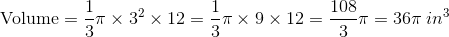# SSAT Upper Level Math : How to find the volume of a cone

## Example Questions

### Example Question #1 : How To Find The Volume Of A Cone

Chestnut wood has a density of about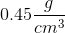. A right circular cone made out of chestnut wood has a height of three meters, and a base with a radius of two meters. What is its mass in kilograms (nearest whole kilogram)?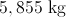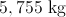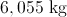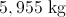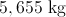Explanation:

First, convert the dimensions to cubic centimeters by multiplying by: the cone has height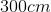, and its base has radius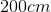.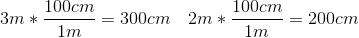Its volume is found by using the formula and the converted height and radius.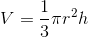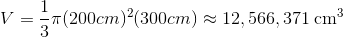Now multiply this byto get the mass.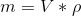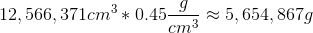Finally, convert the answer to kilograms.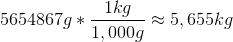### Example Question #2 : How To Find The Volume Of A Cone

A cone has the height of 4 meters and the circular base area of 4 square meters. If we want to fill out the cone with water (density =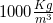), what is the mass of required water (nearest whole kilogram)?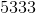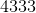6333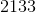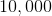Explanation:

The volume of a cone is: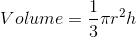whereis the radius of the circular base, andis the height (the perpendicular distance from the base to the vertex).

As the circular base area is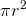, so we can rewrite the volume formula as follows: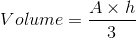whereis the circular base area and known in this problem. So we can write: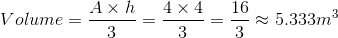We know that density is defined as mass per unit volume or: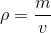Where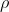is the density;is the mass and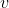is the volume. So we get: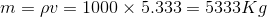### Example Question #3 : How To Find The Volume Of A Cone

The vertical height (or altitude) of a right cone is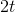. The radius of the circular base of the cone is. Find the volume of the cone in terms of.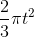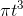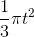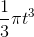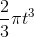Explanation:

The volume of a cone is:whereis the radius of the circular base, andis the height (the perpendicular distance from the base to the vertex).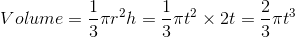### Example Question #4 : How To Find The Volume Of A Cone

A right cone has a volume of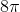, a height of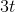and a radius of the circular base of. Find.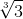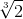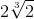Explanation:

The volume of a cone is given by:whereis the radius of the circular base, andis the height; the perpendicular distance from the base to the vertex. Substitute the known values in the formula: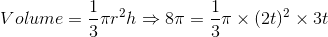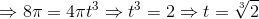### Example Question #5 : How To Find The Volume Of A Cone

A cone has a diameter of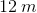and a height of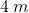. In cubic meters, what is the volume of this cone?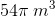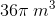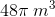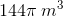Explanation:

First, divide the diameter in half to find the radius.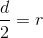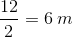Now, use the formula to find the volume of the cone.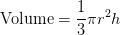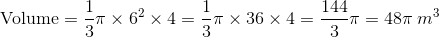### Example Question #6 : How To Find The Volume Of A Cone

A cone has a radius ofinches and a height ofinches. Find the volume of the cone.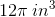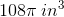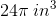Explanation:

The volume of a cone is given by the formula:Now, plug in the values of the radius and height to find the volume of the given cone.